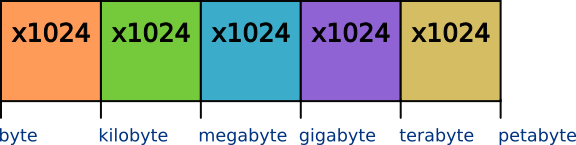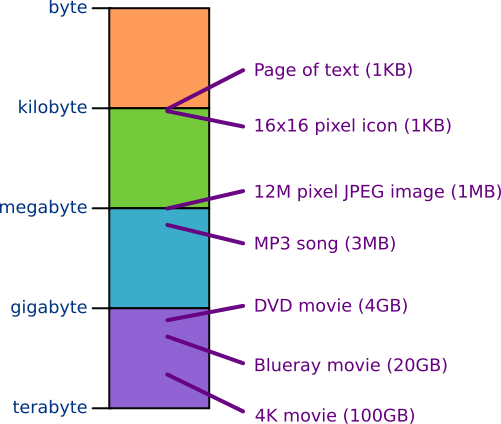# Units of storage

Martin McBride, 2016-11-21
Tags bit byte kilobyte megabyte gigabyte terabyte petabyte
Categories data representation binary numbers

When you buy a new computer, two things you will probably look at is the amount of memory (RAM) it has, and the size of its hard disk. The memory and disk are two types of storage. The amount of storage is measured in bytes.

The memory and disk space of a modern computer is very large, so we don't usually express the amount of space directly in bytes. Instead, we use a more suitable unit of storage - kilobytes, megabytes, gigabytes etc.

We do the same thing with other measurements. For example, you might say a person's height is 1.8 metres, but if you were measuring the distance between two towns, you would state it in kilometres.

## File sizes

When we describe the size of a file, we use the same units of storage - kilobytes, megabytes etc. This allows us to work out how much of our disk space a particular file will use up (or alternatively, how many files of that type will fit onto our disk).

If you are downloading a file from the Internet, and you know how fast your connection is (how many megabytes per second your connection can download), then you can work out approximately how long it will take to download.

## Storage units

A kilobyte (KB) is equal to 1024 bytes.

Typically, kilo means 1000 - a kilogram is 1000 grams, a kilometre is 1000 metres etc. We use 1000 because it is a nice, round power of 10.

However, computers use binary (base 2) to count, so we naturally prefer powers of 2, ie 2, 4, 8, 16, 32, 64, 128, 256, 512, 1024, etc. Of these, 1024 is the closest to 1000, so we define 1 kilobyte as 1024 bytes.

A megabyte (MB) is equal to 1024 kilobytes, or 1048576 bytes. It is approximately a million bytes.

A gigabyte (GB) is equal to 1024 megabytes, or 1,073,741,824 bytes (that is 1024 times 1024 times 1024). It is approximately a billion bytes.

A terabyte (TB) is equal to 1024 gigabytes, or 1,099,511,627,776 bytes (that is 1024 times 1024 times 1024 times 1024). It is approximately a thousand billion bytes.

A petabyte (PB) is equal to 1024 terabytes.## Examples

Here are some examples of typical files sizes:Copyright (c) Axlesoft Ltd 2021